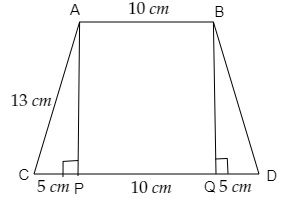# The parallel sides of a trapezium are 20cm and 10cm. Its nonparallel sides are both equal, each being 13cm. Find the area of the trapezium.

Given:

The parallel sides of a trapezium are 20cm and 10cm. Its nonparallel sides are both equal, each being 13cm.

To do:

We have to find the area of the trapezium.

Solution:From the figure,

$\triangle APC$ is a right angled triangle. Therefore, by using Pythagoras theorem,

$AC^2=AP^2+CP^2$ $13^2=AP^2+5^2$

$AP^2=169-25$

$AP=\sqrt{144}$

$AP=12\ cm$

ABCD is a rectangle of length $10\ cm$ and breadth $12\ cm$.

Area of trapezium ABCD$=$ Area of rectangle PQBA$+$ Area of triangle ACP$+$Area of triangle BQD

$=10\times12+\frac{1}{2}\times12\times5+\frac{1}{2}\times12\times5$   ($AP=BQ=12\ cm$)

$=120+30+30\ cm^2$

$=180\ cm^2$

The area of the trapezium is $180\ cm^2$.